# How do we calculate little g?

Of the four known forces of nature, gravity is the strongest on large scales -  it has an ability to lock planets, stars and galaxies in their orbits. On small scales however, gravity appears to act much weaker than its counterparts. Nevertheless, gravity is something we can measure here on Earth.

###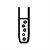How can we measure gravity?

As the apocryphal story goes, Sir Isaac Newton sat beneath an apple tree, when an apple fell onto his head and inspired his work on the theory of gravitation. It is important to note that Newton’s theory is that of the Universal gravitational constant G (also called 'Big G'). His theory describes the force F between two objects of masses m1 and m2 separated by a distance r.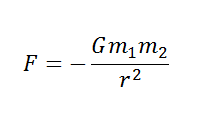When people talk about the gravity of a planet, in general what they mean is the free fall acceleration due to its gravitational field g (which is dependent on the mass that is responsible for the gravitational field) and not (which is a constant). Newton’s work was inspired by Galileo who dropped objects of different masses from the leaning tower of Pisa to show that the time they took to reach the ground is independent of their mass. This tells us that the acceleration due to the gravitational field of Earth must also be the same for all masses. From F = ma = mg, we know it is related to G in the following way,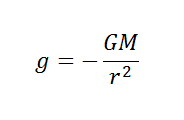On Earth it has a value of g = 9.81 metres per second squared, since G = 0.00000000006674  meters cubed per kilogram per second squared, the mass of Earth = 5,972,000,000,000,000,000,000,000 kilograms and the radius of Earth is = 6,371,000 metres. In the lab, it can be determined by measuring the time (t) it takes for an object to fall from a height (s), using the equations of motion for constant acceleration in a straight line: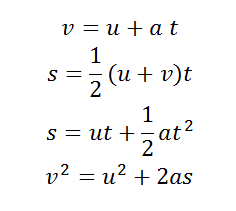Where a is acceleration, u is the initial velocity and v is final velocity.

###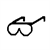How can we improve out answer?

In this experiment the initial velocity u is 0 metres per second, the time can be measured using a stopwatch or light gates, the distance can be measured using a ruler and the variable of interest is the acceleration which in this case is equivalent to g. So you can see that the simplest equation to use to determine g is: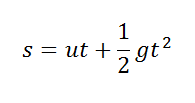And rearranging for g.

If you are having trouble remembering all the equations of motions, one quick check is to make sure that the units on both sides of the equation are the same. On the left hand side, distance is in meters (s[m]), and on the right hand side: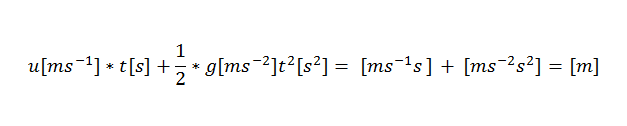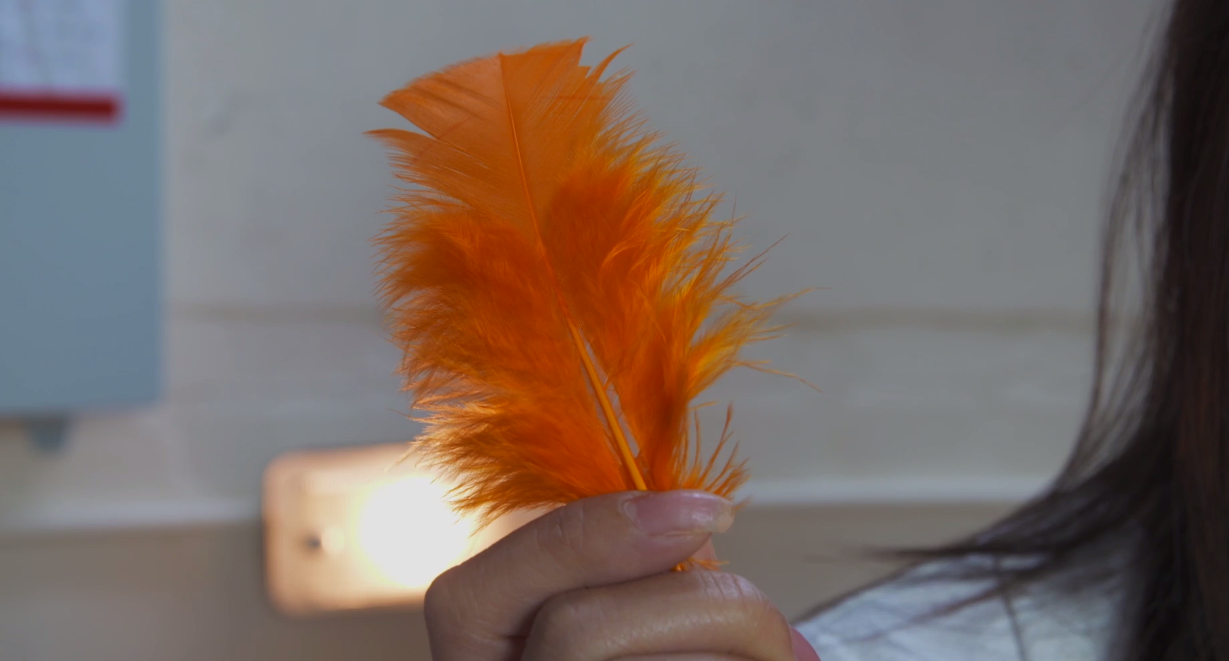So you can see that the units agree.

There are several ways to improve the accuracy for your experiment. Aside from the taking repeat recordings, using light gates instead of a stopwatch and/or an electromagnetic drop (as demonstrated in the video) will reduce the inaccuracies introduced by thinking distance and reaction time. Another thing to consider is increasing the distance of the drop. As this means the uncertainty introduced due to reaction time will be a smaller fraction of the total time it takes to fall. Note that due to the air resistance we experience on Earth, we would not expect to obtain a perfect measurement for g, but using a more streamlined shaped mass such as sphere will work much better than something like a feather.

###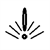How does Newton's theory affect us today?

Newton’s law of universal gravitation successfully predicted the existence of planet Neptune, however there are many other phenomena that Newton’s theory could not explain such as the orbit of Mercury. Newton's theory has since been superseded by Einstein’s theory of general relativity. Einstein’s theory has allowed use GPS satellites to track locations accurately, successfully predicted gravitational lensing (the influence of gravity on light) and more recently gravitational waves (the emission of gravitational radiation).  Unfortunately however it is incompatible with quantum mechanics leading to much current research being focussed on the testing of gravity and new gravity theories.

In general, Newton’s theory of gravity is a good approximation on small scales and is very simple to use. This simple experiment can be done anywhere and in doing it shows your ability to use both analogue and digital apparatus. It demonstrates the importance of human reaction time in error budgeting but also there that there is a lot of room for improvement (for example if you were to redo it in a vacuum, the absence of air resistance will improve the accuracy), if you get the chance it is definitely worth having a play with different object shapes and distances to see how your answer changes.

###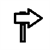Next steps

These links are being provided as a convenience and for informational purposes only; they do not constitute an endorsement or an approval by the University of Birmingham of any of the information contained on external website. The University of Birmingham bears no responsibility for the accuracy, legality or content of the external site or for that of subsequent links. Please contact the external site for answers to questions regarding its content.